Next: What is Voronoi diagram Up: Voronoi Diagram and Delaunay Previous: Voronoi Diagram and Delaunay   Contents

## What is cell complex? What is triangulation?

A cell complex or simply complex in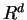is a set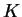of convex polyhedra (called cells) insatisfying two conditions: (1) Every face of a cell is a cell (i.e. in), and (2) If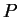and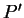are cells, then their intersection is a common face of both. A simplicial complex is a cell complex whose cells are all simplices.

The body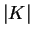of a complexis the union of all cells. When a subsetofis the body of a simplicial complex, thenis said to be a triangulation of. For a finite set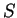of points in, a triangulation ofis a simplicial complexwith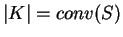.

Komei Fukuda 2004-08-26